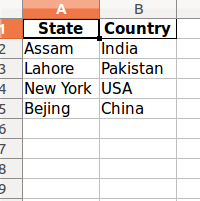Open in App
Not now

# Python | Split given list and insert in excel file

• Last Updated : 28 Feb, 2019

Given a list containing Names and Addresses consecutively, the task is to split these two elements at a time and insert it into excel.

We can use a very popular library for data analysis, Pandas. Using pandas we can easily manipulate the columns and simply insert the filtered elements into excel file using df.to_excel() function.

Below is the implementation :

 `# Python code to split the list two element ``# at a time and insert it into excel.`` ` `# Importing pandas as pd``import` `pandas as pd`` ` `# List initialization``list1 ``=` `[``'Assam'``, ``'India'``,``         ``'Lahore'``, ``'Pakistan'``, ``         ``'New York'``, ``'USA'``,``         ``'Bejing'``, ``'China'``]`` ` `df ``=` `pd.DataFrame()`` ` `# Creating two columns``df[``'State'``] ``=` `list1[``0``::``2``]``df[``'Country'``] ``=` `list1[``1``::``2``]`` ` `# Converting to excel``df.to_excel(``'result.xlsx'``, index ``=` `False``)`

Output :My Personal Notes arrow_drop_up